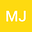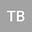B ä cklund Transformation of (3+1)-Dimensional Variable Coefficient Potential-YTSF Equation and Related Problems
••Taogetusang Bao
Inner Mongolia Normal University

Corresponding Author:[email protected]

Author Profile## Abstract

Based on the Bell polynomial method, the (3+1)-dimensional variable coefficient Potential-YTSF equation is transformed into bilinear form, and the double Bell polynomial B a ̵̈ cklund transformation, bilinear B a ̵̈ cklund transformation, Lax pair and infinite conservation law of this equation are constructed. Firstly, the Lax integrability of the equation is proved by the B a ̵̈ cklund transformation of double Bell polynomials, and the infinite conservation law is constructed. Secondly, the exact solution of the equation is obtained by bilinear B a ̵̈ cklund transformation and symbolic computation system Mathematica. Finally, we illustrate their properties by making some graphs of soliton solutions.